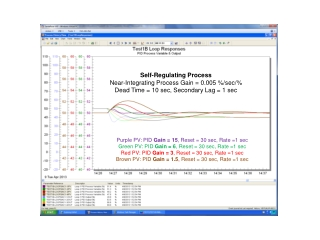DownloadDownload PresentationPurple PV: PID Gain = 15 , Reset = 30 sec, Rate =1 sec

Purple PV: PID Gain = 15 , Reset = 30 sec, Rate =1 sec

Télécharger la présentationPurple PV: PID Gain = 15 , Reset = 30 sec, Rate =1 sec

- - - - - - - - - - - - - - - - - - - - - - - - - - - E N D - - - - - - - - - - - - - - - - - - - - - - - - - - -
Presentation Transcript

1. Self-Regulating Process Near-Integrating Process Gain = 0.005 %/sec/% Dead Time = 10 sec, Secondary Lag = 1 sec Purple PV: PID Gain = 15, Reset = 30 sec, Rate =1 sec Green PV: PID Gain = 6, Reset = 30 sec, Rate =1 sec Red PV: PID Gain = 3, Reset = 30 sec, Rate =1 sec Brown PV: PID Gain = 1.5, Reset = 30 sec, Rate =1 sec

2. Integrating Process True Integrating Process Gain = 0.005 %/sec/% Dead Time = 10 sec, Secondary Lag = 1 sec Purple PV: PID Gain = 15, Reset = 30 sec, Rate =1 sec Green PV: PID Gain = 6, Reset = 30 sec, Rate =1 sec Red PV: PID Gain = 3, Reset = 30 sec, Rate =1 sec Brown PV: PID Gain = 1.5, Reset = 30 sec, Rate =1 sec

3. Runaway Process Near-Integrating Process Gain = 0.005 %/sec/% Dead Time = 10 sec, Secondary Lag = 1 sec Purple PV: PID Gain = 15, Reset = 30 sec, Rate =1 sec Green PV: PID Gain = 6, Reset = 30 sec, Rate =1 sec Red PV: PID Gain = 3, Reset = 30 sec, Rate =1 sec Brown PV: PID Gain = 1.5, Reset = 30 sec, Rate =1 sec

4. To prevent the start of oscillations: To prevent large slow oscillations: Lambda Integrating Process Tuning (lambda = dead time) For Test Cases: Kc= controller gain (dimensionless) Ki= integrating process gain (%/sec/% or 1/sec) Ti= integral (reset) time setting (sec/repeat or sec)